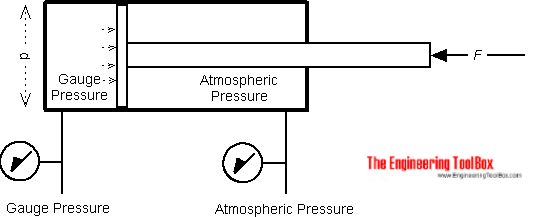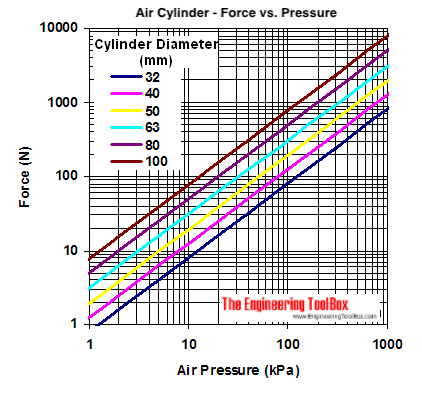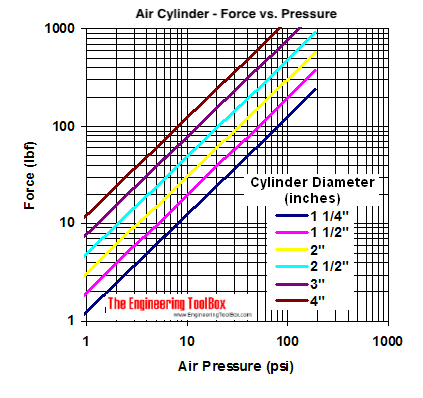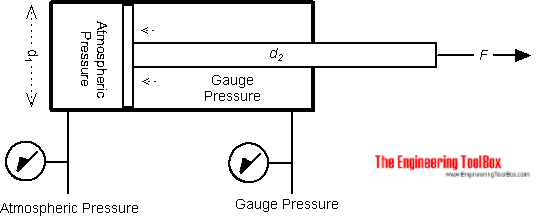Engineering ToolBox - Resources, Tools and Basic Information for Engineering and Design of Technical Applications!

# Pneumatic Cylinder - Excerted Force vs. Pressure

## Force excerted by pneumatic air cylinders vs. air pressure cylinder size - online calculator.

### Single Acting CylinderThe force exerted by a single acting pneumatic cylinder can be expressed as

F = p A

= p π d2 / 4                                           (1)

where

F = force exerted (N)

p = gauge pressure (N/m2, Pa)

A = full bore area (m2)

d = full bore piston diameter (m)

#### Single Acting Cylinder Calculator  - Output Stroke

##### Calculate Force with known Pressure and Diameter

Pressure (kPa) (1 bar = 100 kPa)

Cylinder Diameter (mm)

Force (N)

Pressure (kPa)

Force (N)

Diameter (mm)

#### Example - Single Acting Piston

The force exerted by a single acting pneumatic cylinder with 1 bar (105 N/m2) and full bore diameter of 100 mm (0.1 m) can be calculated as

F = p π d2 / 4

= (105 N/m2) π (0.1 m)2 / 4

= 785 N

= 0.785 kN

### Air Cylinder - Pressure/Force Diagram

#### SI-units#### Imperial Units### Double Acting CylinderThe force exerted by double acting pneumatic cylinder on outstroke can be expressed as (1). The force exerted on instroke can be expressed as

F = p π (d12 - d22) / 4                                     (2)

where

d1 = full bore piston diameter (m)

d2 = piston rod diameter (m)

#### Double Acting Cylinder Calculator  - Input Stroke

##### Calculate Force with known Pressure and Diameter

Pressure (kPa)

Cylinder Diameter (mm)

Piston Rod Diameter (mm)

##### Calculate required Diameter with known Force and Pressure

Force (N)

Pressure (kPa)

Piston Rod Diameter (mm)

##### Calculate required Pressure with known Force and Diameter

Force (N)

Diameter (mm)

Piston Rod Diameter (mm)

#### Example - Double Acting Piston

The force exerted from a single acting pneumatic cylinder with 1 bar (105 N/m2), full bore diameter of 100 mm (0.1 m) and rod diameter 10 mm (0.01 m) can be calculated as

F = p π (d12 - d22) / 4

= (105 N/m2) π [(0.1 m)2 - (0.01 m)2] / 4

= 778 N

= 0.78 kN

• instroke capacity is reduced compared to outstroke capacity - due to the rod and reduced active pressurized areal

## Engineering ToolBox - SketchUp Extension - Online 3D modeling!

Add standard and customized parametric components - like flange beams, lumbers, piping, stairs and more - to your Sketchup model with the Engineering ToolBox - SketchUp Extension - enabled for use with the amazing, fun and free SketchUp Make and SketchUp Pro .Add the Engineering ToolBox extension to your SketchUp from the SketchUp Pro Sketchup Extension Warehouse!

Translate

## Privacy

We don't collect information from our users. Only emails and answers are saved in our archive. Cookies are only used in the browser to improve user experience.

Some of our calculators and applications let you save application data to your local computer. These applications will - due to browser restrictions - send data between your browser and our server. We don't save this data.

## Citation

• Engineering ToolBox, (2008). Pneumatic Cylinder - Excerted Force vs. Pressure. [online] Available at: https://www.engineeringtoolbox.com/pneumatic-cylinder-force-d_1273.html [Accessed Day Mo. Year].

Modify access date.

. .

#### Scientific Online Calculator6 24